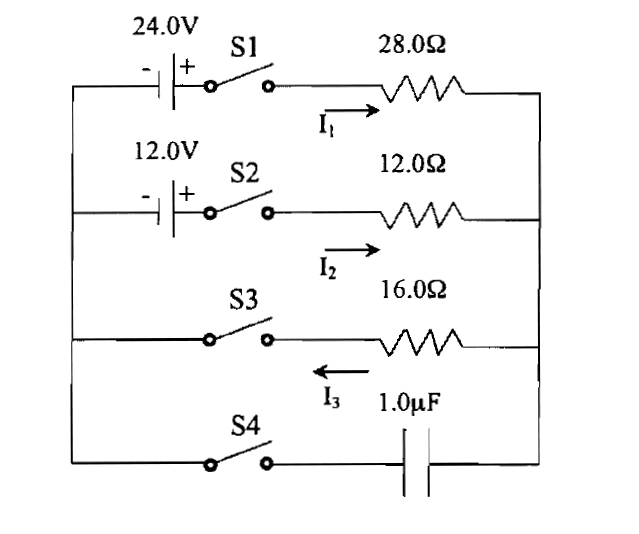# Kirchhoff's voltage law problem: Batteries, switches, resistors

• kenok1216

## Homework Statementfor S1,S2,S3,S4 are all closed at the long time
find the current in each resistor

## The Attempt at a Solution

why I3 is not = i2?
the solution of this question is
24-28I1-I212-12=0
7I1-32=3-----(1)

12-12I2-163=0
3I2+43=3 ---(2)

I1+I2-I3=0---(3)

I1=0.492 I2=0.148 I3=0.639[/B]
why there are 3 current in 2loop and I2 is not =I3 and why at the top loop
the equation is not 24-28I1-[I1-I2]12-12=0?